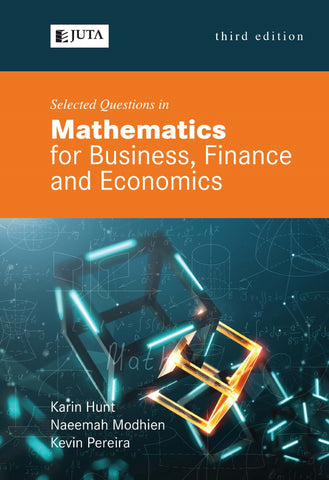## Selected Questions in Mathematics for Business, Finance and Economics 3e (Print)

• R 618.00
• Save R 47

This exercise book is comprised of questions covering topics in Differential and Integral Calculus, Matrix Algebra and Linear Programming. The aim of the work is to develop student competency in using mathematical techniques as a “toolbox” for the solution of problems relevant to Economics, Business and Finance. The book provides an introductory revision chapter of basic mathematical principles followed by chapters of multiple-choice questions each covering a particular section of the work. In addition to multiple-choice questions, there are extension tutorials requiring written responses and sample tests covering each section of the work. Answers are provided to each question at the back of the book together with a formula sheet for easy reference.

Key words that describe the book:

• Algebraic manipulation
• Mathematical techniques
• Interpretation of mathematical concepts
• Problem-solving

We Also Recommend